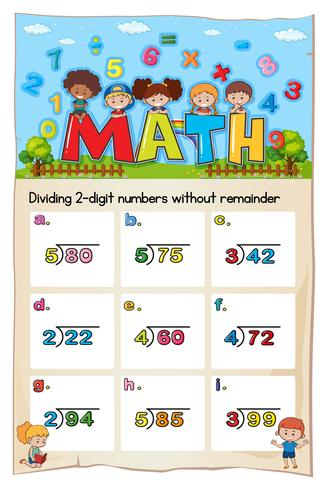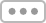Explore
All Vectors

# Math worksheet template for dividing two digitsMath worksheet template for dividing two digits illustration